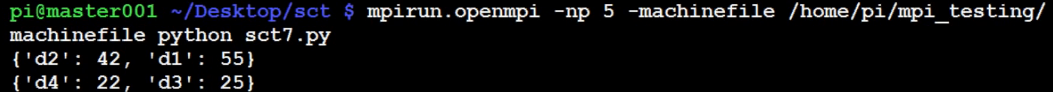## Message and data tagging for send and recv MPI commands tutorial

### MPI send and recv "tag" parameter

```from mpi4py import MPI

comm = MPI.COMM_WORLD
rank=comm.rank
size=comm.size
name=MPI.Get_processor_name()

if rank == 0:
shared = {'d1':55,'d2':42}
comm.send(shared, dest=1)

if rank == 1:
receive = comm.recv(source=0)
print receive
print receive['d1']

```

So this isn't tagging just yet, but I wanted to show you this. What is so special about this? Well, the data we're sending here is not just an int or a string, this is a dictionary! So, as you can see, we can also send objects with MPI4py. This is actually pretty exciting, at least to me.

```from mpi4py import MPI

comm = MPI.COMM_WORLD
rank=comm.rank
size=comm.size
name=MPI.Get_processor_name()

if rank == 0:
shared = {'d1':55,'d2':42}
comm.send(shared, dest=1, tag=1)

shared2 = {'d3':25,'d4':22}
comm.send(shared2, dest=1, tag=2)

if rank == 1:
receive = comm.recv(source=0, tag=1)
print receive
receive2 = comm.recv(source=0, tag=2)
print receive2
```

Tagging messages is basically a requirement, unless maybe you treat a node itself as a variable name, and that node only produces one element. The idea of tagging is that you can actually put a label to the data that you're sending, otherwise there's really no way to differentiate between one bit of "data" and another.

Tagging allows us to control data flow, and make sure correct data gets stored where we expect. As you can see, we use the tag parameter in both

`mpirun.openmpi -np 5 -machinefile /home/pi/mpi_testing/machinefile python ~/Desktop/sct/sct7.py`The next tutorial:• Build a Supercomputer with Raspberry Pis

• Intro

• Supplies

• Installing Operating System

• Downloading and installing MPI

• Testing Supercomputer

• MPI with MPI4py Introduction

• Installing mpi4py for use with Python and MPI

• First basic MPI script with mpi4py

• Using conditional, Python, statements alongside MPI commands example

• Getting network processor size with the size command

• Sending and Receiving data using send and recv commands with MPI

• Dynamically sending messages to and from processors with MPI and mpi4py

• Message and data tagging for send and recv MPI commands tutorial
• MPI broadcasting tutorial with Python, mpi4py, and bcast

• Scatter with MPI tutorial with mpi4py

• Gather command with MPI, mpi4py, and Python